## T100——q查询，子母查询（汇总——明细）练习笔记 转

o
osc_mervd488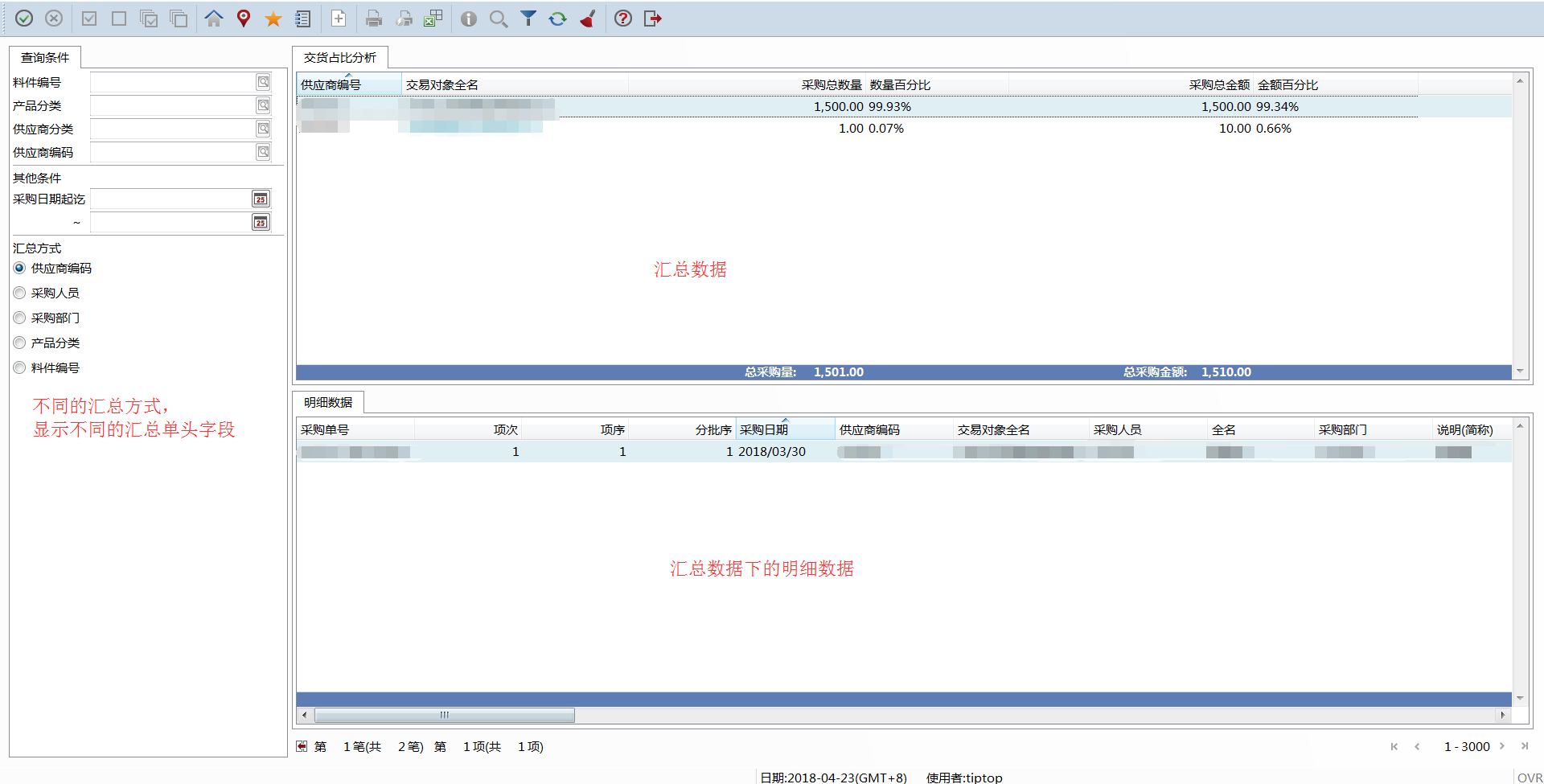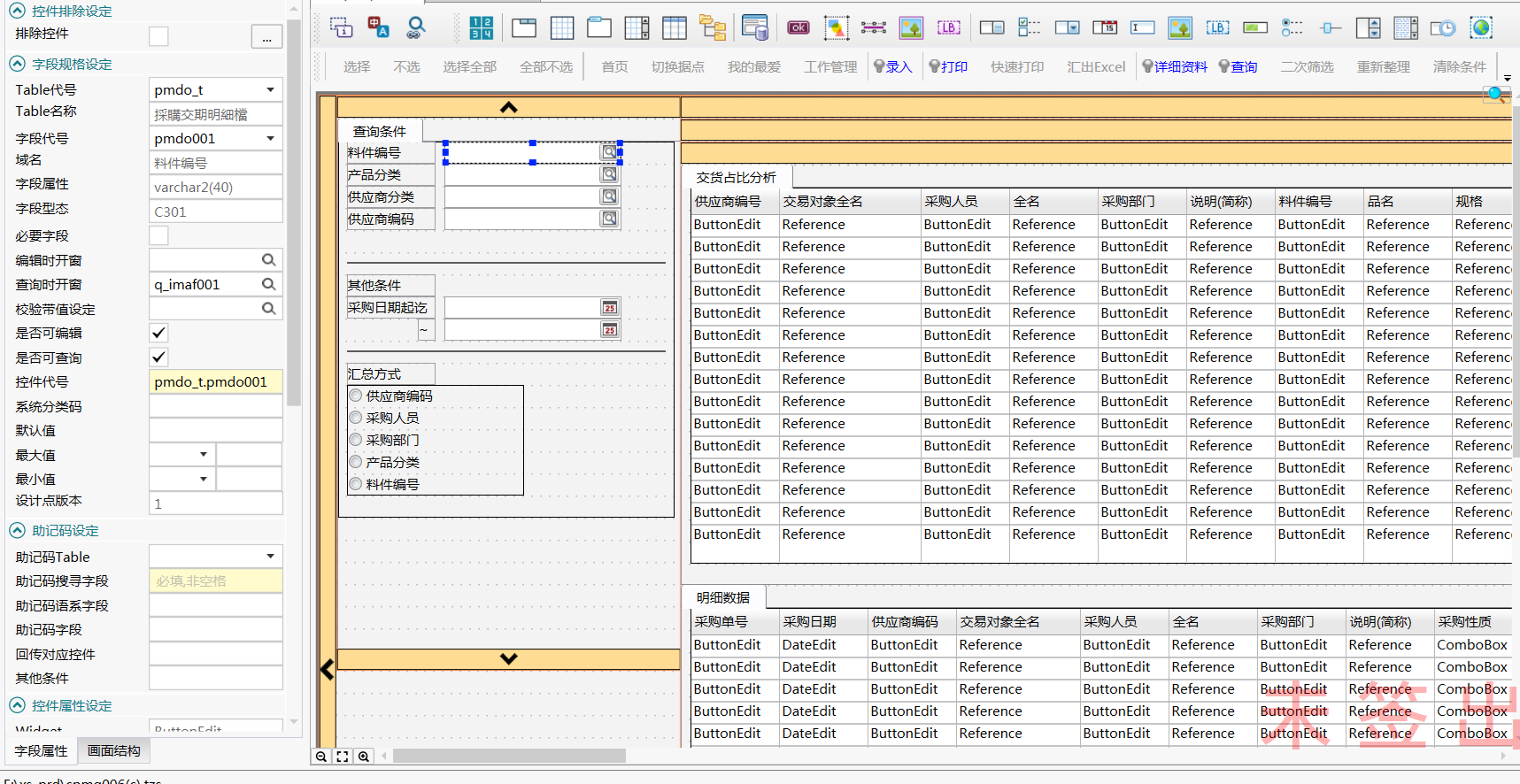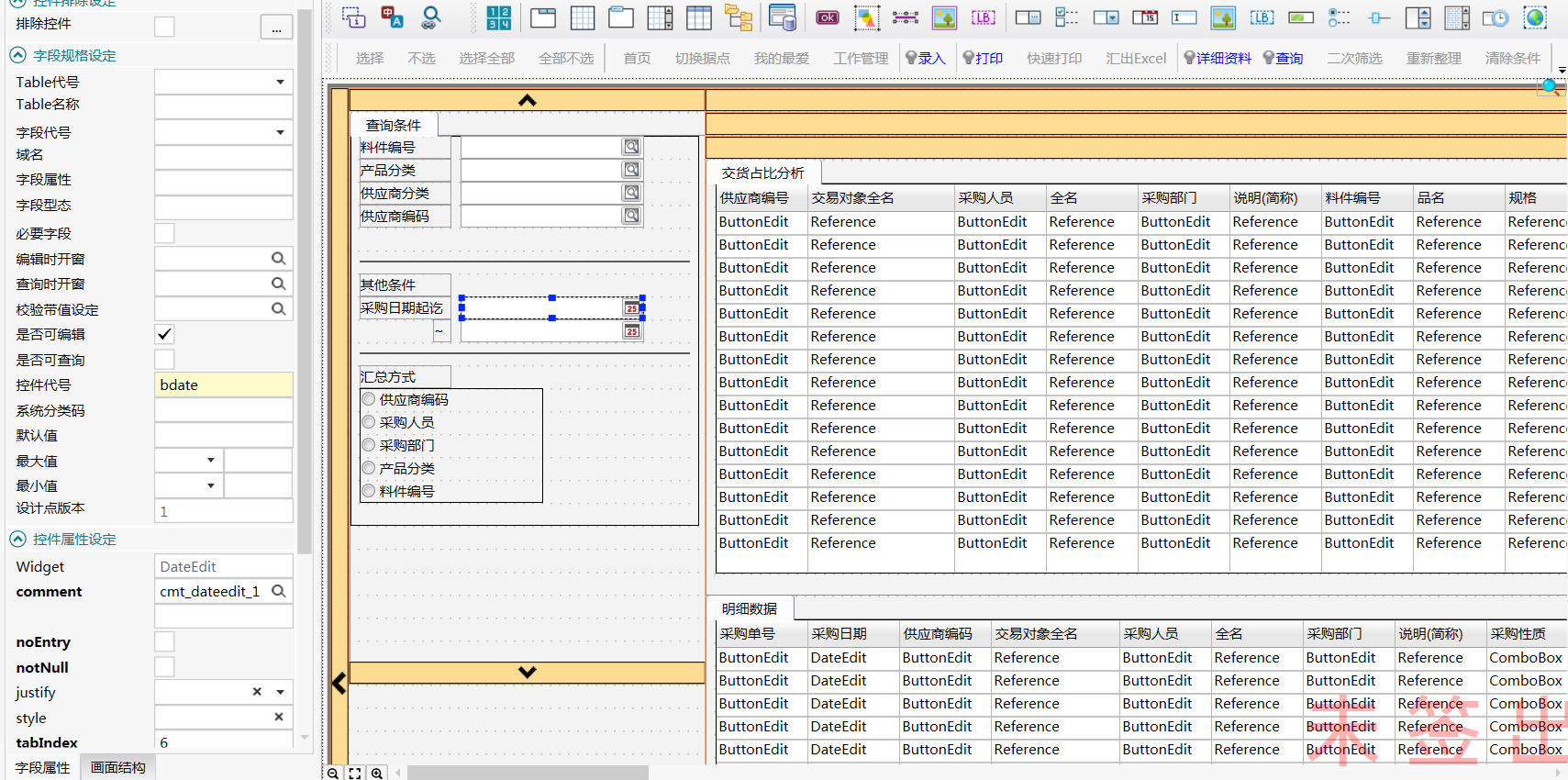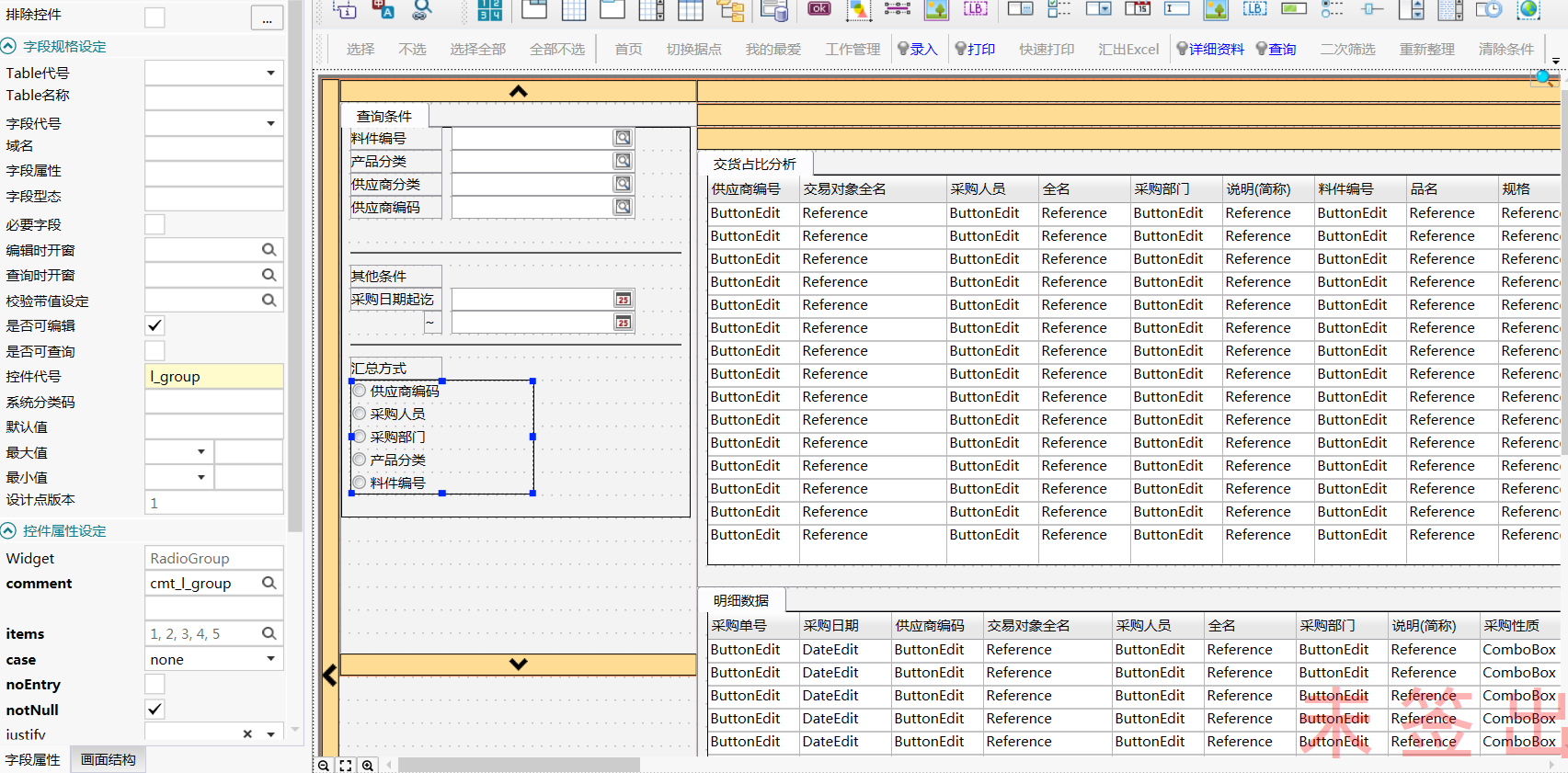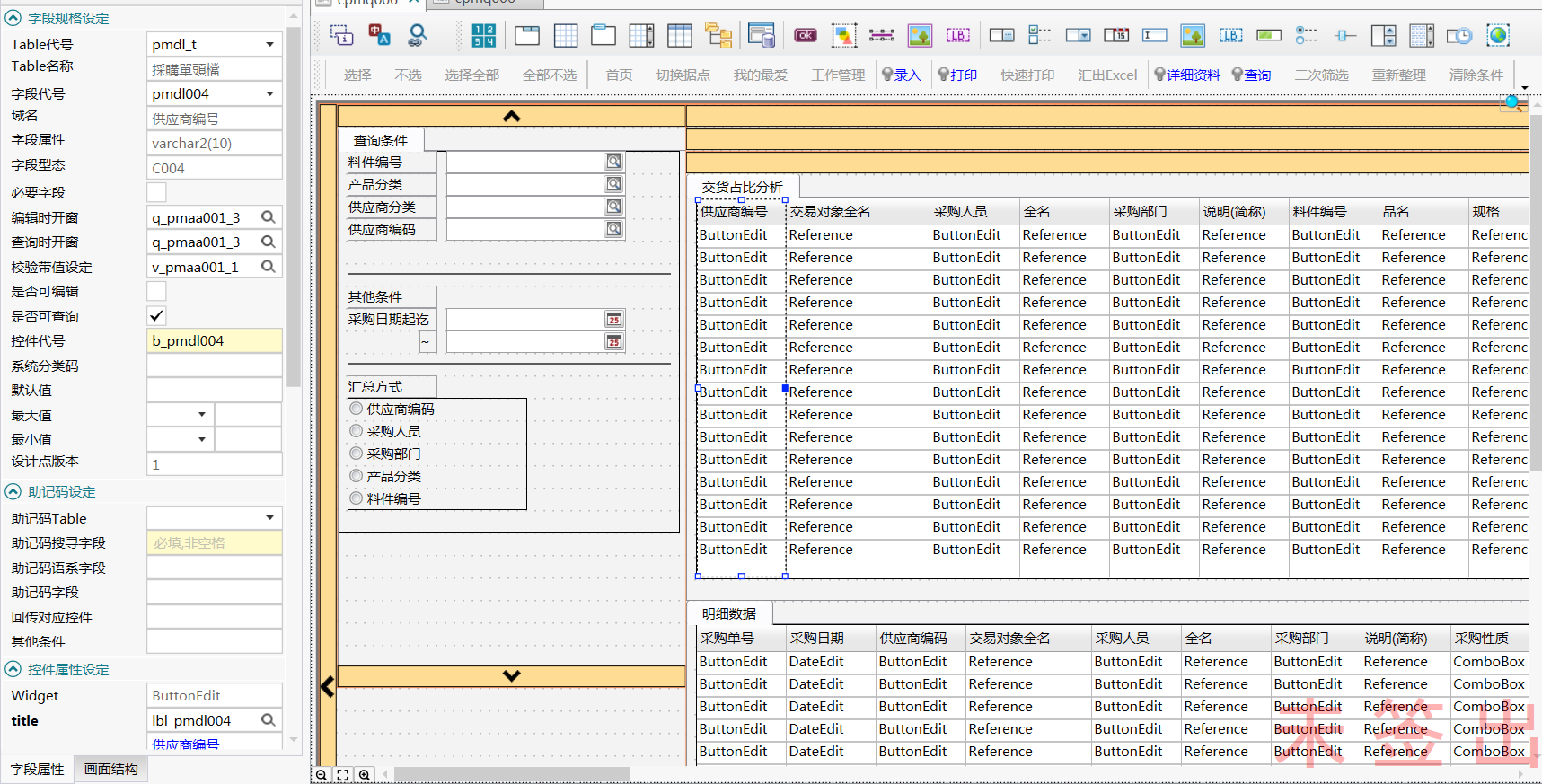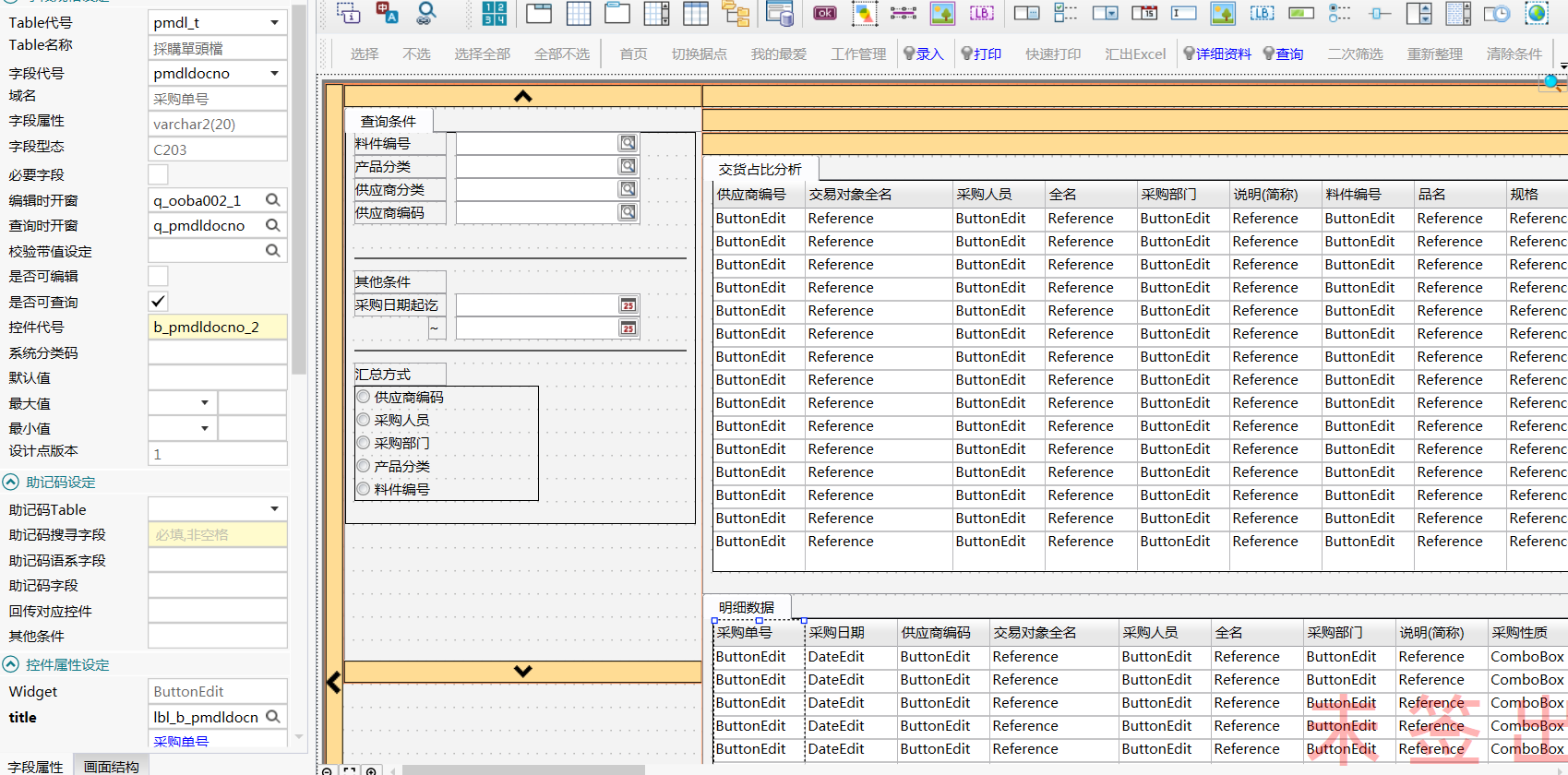``````#add-point:input段落 name="ui_dialog.input"
INPUT BY NAME g_master.bdate,g_master.edate,g_master.l_group
ATTRIBUTE(WITHOUT DEFAULTS)

AFTER FIELD edate
IF NOT cl_null(g_master.edate) AND NOT cl_null(g_master.bdate) THEN
IF g_master.edate < g_master.bdate THEN
LET g_master.edate = NULL
DISPLAY g_master.edate TO edate
NEXT FIELD edate
END IF
END IF

END INPUT
``````

``````#add-point:construct段落 name="ui_dialog.construct"
CONSTRUCT BY NAME g_master.wc ON pmdo001,imaa009,pmaa080,pmdl004

ON ACTION controlp INFIELD pmdo001
INITIALIZE g_qryparam.* TO NULL
LET g_qryparam.state = 'c'
LET g_qryparam.reqry = FALSE
CALL q_imaf001()                           #呼叫開窗
DISPLAY g_qryparam.return1 TO pmdo001  #顯示到畫面上
NEXT FIELD pmdo001                     #返回原欄位

ON ACTION controlp INFIELD imaa009
INITIALIZE g_qryparam.* TO NULL
LET g_qryparam.state = 'c'
LET g_qryparam.reqry = FALSE
CALL q_rtax001()                           #呼叫開窗
DISPLAY g_qryparam.return1 TO imaa009  #顯示到畫面上
NEXT FIELD imaa009                     #返回原欄位

ON ACTION controlp INFIELD pmaa080
INITIALIZE g_qryparam.* TO NULL
LET g_qryparam.state = 'c'
LET g_qryparam.reqry = FALSE
LET g_qryparam.arg1 = '251'
CALL q_oocq002()                           #呼叫開窗
DISPLAY g_qryparam.return1 TO pmaa080  #顯示到畫面上
NEXT FIELD pmaa080                     #返回原欄位

ON ACTION controlp INFIELD pmdl004
INITIALIZE g_qryparam.* TO NULL
LET g_qryparam.state = 'c'
LET g_qryparam.reqry = FALSE
CALL q_pmaa001_3()                           #呼叫開窗
DISPLAY g_qryparam.return1 TO pmdl004  #顯示到畫面上
NEXT FIELD pmdl004                     #返回原欄位

END CONSTRUCT
``````

``````#add-point:page1自定義行為 name="ui_dialog.body.page1.action"
ON ACTION modify_detail
LET l_wc_web = l_wc
LET l_wc_web = cl_replace_str(l_wc_web,"pmdo001","imaa001")
LET l_wc_web = cl_replace_str(l_wc_web,"pmdl004","pmaa001")
CASE g_master.l_group
WHEN '1'
CALL apmr006_x01(l_wc_web)
WHEN '2'
CALL apmr006_x02(l_wc_web)
WHEN '3'
CALL apmr006_x03(l_wc_web)
WHEN '4'
CALL apmr006_x04(l_wc_web)
WHEN '5'
CALL apmr006_x05(l_wc_web)
END CASE
``````

``````#+ 單身陣列填充
PRIVATE FUNCTION cpmq006_b_fill()

DEFINE ls_wc           STRING
DEFINE l_pid           LIKE type_t.chr50
DEFINE ls_sql_rank     STRING

CALL cl_set_comp_visible("b_pmdl004,b_pmdl004_desc,b_pmdl002,b_pmdl002_desc,b_pmdl003,b_pmdl003_desc,b_pmdo001,b_pmdo001_desc,b_pmdo001_desc_1,b_imaa009,b_imaa009_desc",FALSE)
CASE g_master.l_group
WHEN '1'    #供应商编码
CALL cl_set_comp_visible("b_pmdl004,b_pmdl004_desc",TRUE)
WHEN '2'    #采购人员
CALL cl_set_comp_visible("b_pmdl002,b_pmdl002_desc",TRUE)
WHEN '3'    #采购部门
CALL cl_set_comp_visible("b_pmdl003,b_pmdl003_desc",TRUE)
WHEN '4'    #产品分类
CALL cl_set_comp_visible("b_imaa009,b_imaa009_desc",TRUE)
WHEN '5'    #料件编号
CALL cl_set_comp_visible("b_pmdo001,b_pmdo001_desc,b_pmdo001_desc_1",TRUE)
END CASE

IF cl_null(g_master.wc) THEN
LET g_master.wc = " pmdlsite = '",g_site CLIPPED,"' AND pmdlent = '",g_enterprise CLIPPED,"' "
ELSE
LET g_master.wc = g_master.wc CLIPPED," AND pmdlsite = '",g_site CLIPPED,"' AND pmdlent = '",g_enterprise CLIPPED,"' "
END IF

LET g_master.wc = g_master.wc CLIPPED," AND (pmdl005 = '1' OR pmdl005 = '2' OR pmdl005 = '3' OR pmdl005 = '5' ) AND pmdlstus='Y'"

IF NOT cl_null(g_master.bdate) THEN
LET g_master.wc = g_master.wc , " AND pmdldocdt >= to_date( '",g_master.bdate,"','yy/mm/dd') "
END IF

IF NOT cl_null(g_master.edate) THEN
LET g_master.wc = g_master.wc , " AND pmdldocdt <= to_date('",g_master.edate,"','yy/mm/dd') "
END IF

LET l_wc = g_master.wc

IF cl_null(g_wc_filter) THEN
LET g_wc_filter = " 1=1"
END IF
IF cl_null(g_wc) THEN
LET g_wc = " 1=1"
END IF
IF cl_null(g_wc2) THEN
LET g_wc2 = " 1=1"
END IF

LET ls_wc = g_wc, " AND ", g_wc2, " AND ", g_wc_filter

CALL g_pmdl_d.clear()

LET g_cnt = l_ac
IF g_cnt = 0 THEN
LET g_cnt = 1
END IF
LET l_ac = 1

# b_fill段sql組成及FOREACH撰寫
#應用 qs04 樣板自動產生(Version:9)
#+ b_fill段資料取得(包含sql組成及FOREACH段撰寫)
LET ls_sql_rank = "SELECT  UNIQUE pmdl004,'',pmdl002,'',pmdl003,'','','','','','','','','',''  ,DENSE_RANK() OVER( ORDER BY pmdl_t.pmdldocno) AS RANK FROM pmdl_t",

#table2
" LEFT JOIN pmdn_t ON pmdnent = pmdlent AND pmdldocno = pmdndocno",

"",
" WHERE pmdlent= ? AND 1=1 AND ", ls_wc
" ORDER BY pmdl_t.pmdldocno"

LET ls_sql_rank = " SELECT pmdl004,pmaal003,pmdl002,ooag011,pmdl003,ooefl003,pmdo001,imaal003,imaal004,imaa009,rtaxl003,pmdo006,pmdo033 ",
" FROM pmdo_t ",
" LEFT JOIN pmdn_t ON pmdnent=pmdoent AND pmdndocno=pmdodocno AND pmdnseq=pmdoseq  ",
" LEFT JOIN pmdl_t ON pmdlent=pmdnent AND pmdldocno=pmdndocno ",
" LEFT JOIN imaa_t ON imaaent=pmdnent AND imaa001=pmdn001 ",
" LEFT JOIN pmaa_t ON pmaaent=pmdlent AND pmaa001=pmdl004 ",
" LEFT JOIN pmaal_t ON pmaalent=pmdlent AND pmaal001=pmdl004 AND pmaal002='zh_CN' ",
" LEFT JOIN ooag_t ON ooagent=pmdlent AND ooag001=pmdl002 ",
" LEFT JOIN ooefl_t ON ooeflent=pmdlent AND ooefl001=pmdl003 AND ooefl002='zh_CN' ",
" LEFT JOIN imaal_t ON imaaent=pmdnent AND imaal001=pmdn001 AND imaal002='zh_CN' ",
" LEFT JOIN rtaxl_t ON rtaxlent=imaaent AND rtaxl001=imaa009 AND rtaxl002='zh_CN' ",
" WHERE  ",l_wc CLIPPED
{

LET g_sql = "SELECT COUNT(1) FROM (",ls_sql_rank,")"

PREPARE b_fill_cnt_pre FROM g_sql  #總筆數
EXECUTE b_fill_cnt_pre USING g_enterprise INTO g_tot_cnt
FREE b_fill_cnt_pre

}
LET g_sql = "SELECT COUNT(1) FROM (",ls_sql_rank,")"

PREPARE b_fill_cnt_pre FROM g_sql  #總筆數
EXECUTE b_fill_cnt_pre  INTO g_tot_cnt
FREE b_fill_cnt_pre

CASE g_detail_page_action
WHEN "detail_first"
LET g_pagestart = 1

WHEN "detail_previous"
LET g_pagestart = g_pagestart - g_num_in_page
IF g_pagestart < 1 THEN
LET g_pagestart = 1
END IF

WHEN "detail_next"
LET g_pagestart = g_pagestart + g_num_in_page
IF g_pagestart > g_tot_cnt THEN
LET g_pagestart = g_tot_cnt - (g_tot_cnt mod g_num_in_page) + 1
WHILE g_pagestart > g_tot_cnt
LET g_pagestart = g_pagestart - g_num_in_page
END WHILE
END IF

WHEN "detail_last"
LET g_pagestart = g_tot_cnt - (g_tot_cnt mod g_num_in_page) + 1
WHILE g_pagestart > g_tot_cnt
LET g_pagestart = g_pagestart - g_num_in_page
END WHILE

OTHERWISE
LET g_pagestart = 1

END CASE

LET g_sql = "SELECT pmdl004,'',pmdl002,'',pmdl003,'','','','','','','','','',''",
" FROM (",ls_sql_rank,")",
" WHERE RANK >= ",g_pagestart,
" AND RANK < ",g_pagestart + g_num_in_page

LET g_sql = " WITH t1 AS ( ",
" SELECT pmdl004,pmaal003,pmdl002,ooag011,pmdl003,ooefl003,pmdo001,imaal003,imaal004,imaa009,rtaxl003,pmdo006,pmdo033 ",
" FROM pmdo_t ",
" LEFT JOIN pmdn_t ON pmdnent=pmdoent AND pmdndocno=pmdodocno AND pmdnseq=pmdoseq  ",
" LEFT JOIN pmdl_t ON pmdlent=pmdnent AND pmdldocno=pmdndocno ",
" LEFT JOIN imaa_t ON imaaent=pmdnent AND imaa001=pmdn001 ",
" LEFT JOIN pmaa_t ON pmaaent=pmdlent AND pmaa001=pmdl004 ",
" LEFT JOIN pmaal_t ON pmaalent=pmdlent AND pmaal001=pmdl004 AND pmaal002='zh_CN' ",
" LEFT JOIN ooag_t ON ooagent=pmdlent AND ooag001=pmdl002 ",
" LEFT JOIN ooefl_t ON ooeflent=pmdlent AND ooefl001=pmdl003 AND ooefl002='zh_CN' ",
" LEFT JOIN imaal_t ON imaaent=pmdnent AND imaal001=pmdn001 AND imaal002='zh_CN' ",
" LEFT JOIN rtaxl_t ON rtaxlent=imaaent AND rtaxl001=imaa009 AND rtaxl002='zh_CN' ",
" WHERE pmdoent=? AND  ",l_wc CLIPPED," ) "

CASE g_master.l_group
WHEN '1'    #供应商编码
LET g_sql = g_sql, " SELECT pmdl004,pmaal003,'','','','','','','','','',SUM(pmdo006),to_char(SUM(pmdo006)/(SELECT SUM(pmdo006) FROM t1)*100,'fm990.99')||'%',SUM(pmdo033),to_char(SUM(pmdo033)/(SELECT SUM(pmdo033) FROM t1)*100,'fm990.99')||'%' ",
" FROM t1 ",
" GROUP BY pmdl004,pmaal003 ",
" ORDER BY pmdl004 "
WHEN '2'    #采购人员
LET g_sql = g_sql, " SELECT '','',pmdl002,ooag011,'','','','','','','',SUM(pmdo006),to_char(SUM(pmdo006)/(SELECT SUM(pmdo006) FROM t1)*100,'fm990.99')||'%',SUM(pmdo033),to_char(SUM(pmdo033)/(SELECT SUM(pmdo033) FROM t1)*100,'fm990.99')||'%' ",
" FROM t1 ",
" GROUP BY pmdl002,ooag011 ",
" ORDER BY pmdl002 "
WHEN '3'    #采购部门
LET g_sql = g_sql, " SELECT '','','','',pmdl003,ooefl003,'','','','','',SUM(pmdo006),to_char(SUM(pmdo006)/(SELECT SUM(pmdo006) FROM t1)*100,'fm990.99')||'%',SUM(pmdo033),to_char(SUM(pmdo033)/(SELECT SUM(pmdo033) FROM t1)*100,'fm990.99')||'%' ",
" FROM t1 ",
" GROUP BY pmdl003,ooefl003 ",
" ORDER BY pmdl003 "
WHEN '4'    #产品分类
LET g_sql = g_sql, " SELECT '','','','','','','','','',imaa009,rtaxl003,SUM(pmdo006),to_char(SUM(pmdo006)/(SELECT SUM(pmdo006) FROM t1)*100,'fm990.99')||'%',SUM(pmdo033),to_char(SUM(pmdo033)/(SELECT SUM(pmdo033) FROM t1)*100,'fm990.99')||'%' ",
" FROM t1 ",
" GROUP BY imaa009,rtaxl003 ",
" ORDER BY imaa009 "
WHEN '5'    #料件编号
LET g_sql = g_sql, " SELECT '','','','','','',pmdo001,imaal003,imaal004,'','',SUM(pmdo006),to_char(SUM(pmdo006)/(SELECT SUM(pmdo006) FROM t1)*100,'fm990.99')||'%',SUM(pmdo033),to_char(SUM(pmdo033)/(SELECT SUM(pmdo033) FROM t1)*100,'fm990.99')||'%' ",
" FROM t1 ",
" GROUP BY pmdo001,imaal003,imaal004 ",
" ORDER BY pmdo001 "
END CASE

PREPARE cpmq006_pb FROM g_sql
DECLARE b_fill_curs CURSOR FOR cpmq006_pb

OPEN b_fill_curs USING g_enterprise

FOREACH b_fill_curs INTO g_pmdl_d[l_ac].pmdl004,g_pmdl_d[l_ac].pmdl004_desc,g_pmdl_d[l_ac].pmdl002,
g_pmdl_d[l_ac].pmdl002_desc,g_pmdl_d[l_ac].pmdl003,g_pmdl_d[l_ac].pmdl003_desc,g_pmdl_d[l_ac].pmdo001,
g_pmdl_d[l_ac].pmdo001_desc,g_pmdl_d[l_ac].pmdo001_desc_1,g_pmdl_d[l_ac].imaa009,g_pmdl_d[l_ac].imaa009_desc,
g_pmdl_d[l_ac].l_sum_cnt,g_pmdl_d[l_ac].l_sum_cntb,g_pmdl_d[l_ac].l_sum_money,g_pmdl_d[l_ac].l_sum_moneyb

IF SQLCA.sqlcode THEN
INITIALIZE g_errparam TO NULL
LET g_errparam.extend = "FOREACH:"
LET g_errparam.code   = SQLCA.sqlcode
LET g_errparam.popup  = TRUE
CALL cl_err()

EXIT FOREACH
END IF

CALL cpmq006_detail_show("'1'")

IF l_ac > g_max_rec THEN
IF g_error_show = 1 THEN
INITIALIZE g_errparam TO NULL
LET g_errparam.extend =  ""
LET g_errparam.code   =  9035
LET g_errparam.popup  = TRUE
CALL cl_err()

END IF
EXIT FOREACH
END IF
LET l_ac = l_ac + 1

END FOREACH

#應用 qs05 樣板自動產生(Version:3)
#+ b_fill段其他table資料取得(包含sql組成及資料填充)

CALL g_pmdl_d.deleteElement(g_pmdl_d.getLength())

LET g_error_show = 0

LET g_detail_cnt = g_pmdl_d.getLength()
LET l_ac = g_cnt
LET g_cnt = 0

#應用 qs06 樣板自動產生(Version:3)
#+ b_fill段CURSOR釋放
CLOSE b_fill_curs
FREE cpmq006_pb

#調整單身index指標，避免翻頁後指到空白筆數
CALL cpmq006_detail_index_setting()

#重新計算單身筆數並呈現
CALL cpmq006_detail_action_trans()

LET l_ac = 1
IF g_pmdl_d.getLength() > 0 THEN
CALL cpmq006_b_fill2()
END IF

CALL cpmq006_filter_show('pmdl004','b_pmdl004')
CALL cpmq006_filter_show('pmdl002','b_pmdl002')
CALL cpmq006_filter_show('pmdl003','b_pmdl003')
CALL cpmq006_filter_show('pmdo001','b_pmdo001')
CALL cpmq006_filter_show('imaa009','b_imaa009')
CALL cpmq006_filter_show('pmdlstus','b_pmdlstus')

END FUNCTION
``````

``````#+ 單身陣列填充2
PRIVATE FUNCTION cpmq006_b_fill2()

DEFINE li_ac           LIKE type_t.num10

LET li_ac = l_ac

#單身組成
#應用 qs07 樣板自動產生(Version:6)
#+ b_fill2段table資料取得(包含sql組成及資料填充)
#Page2
CALL g_pmdl2_d.clear()

{

#table2
#為避免影響執行效能，若是按上下筆就不重組SQL
IF g_action_choice <> "fetch" OR cl_null(g_action_choice) THEN
LET g_sql = "SELECT  UNIQUE '','','','','','','','','','','','','','','','','','','','','','',
'','','','','','','','','','',pmdnud001,pmdn015,pmdn045,pmdn049,'',pmdn051,'' FROM pmdn_t",

"",
" WHERE pmdnent=? AND pmdndocno=?"

IF NOT cl_null(g_wc2_table2) THEN
LET g_sql = g_sql CLIPPED," AND ",g_wc2_table2 CLIPPED
END IF

LET g_sql = g_sql, " ORDER BY pmdn_t.pmdnseq"

}
LET g_sql = " SELECT pmdldocno,pmdldocdt,pmdl004,pmaal003,pmdl002,ooag011,pmdl003,ooefl003,pmdl005,pmdlud002,pmdlstus,pmdoseq, ",
" pmdoseq1,pmdoseq2,pmdo003,pmdo001,imaal003,imaal004,pmdo004,pmdo005,pmdo006,pmdo011,pmdo012,pmdo013,pmdo009, ",
" pmdo015,pmdo016,pmdo017,pmdo040,pmdo019,pmdo024,pmdo033,pmdnud001,pmdn015,pmdn045,pmdn049,oq1.oocql004,pmdn051,oq2.oocql004 ",
" FROM pmdo_t ",
" LEFT JOIN pmdn_t ON pmdnent=pmdoent AND pmdndocno=pmdodocno AND pmdnseq=pmdoseq  ",
" LEFT JOIN pmdl_t ON pmdlent=pmdnent AND pmdldocno=pmdndocno ",
" LEFT JOIN imaa_t ON imaaent=pmdnent AND imaa001=pmdn001 ",
" LEFT JOIN pmaa_t ON pmaaent=pmdlent AND pmaa001=pmdl004 ",
" LEFT JOIN pmaal_t ON pmaalent=pmdlent AND pmaal001=pmdl004 AND pmaal002='zh_CN' ",
" LEFT JOIN ooag_t ON ooagent=pmdlent AND ooag001=pmdl002 ",
" LEFT JOIN ooefl_t ON ooeflent=pmdlent AND ooefl001=pmdl003 AND ooefl002='zh_CN' ",
" LEFT JOIN imaal_t ON imaaent=pmdnent AND imaal001=pmdn001 AND imaal002='zh_CN' ",
" LEFT JOIN rtaxl_t ON rtaxlent=imaaent AND rtaxl001=imaa009 AND rtaxl002='zh_CN' ",
" LEFT JOIN oocql_t oq1 ON oq1.oocqlent=pmdnent AND oq1.oocql001='265' AND oq1.oocql002=pmdn049 AND oq1.oocql003='zh_CN'  ",
" LEFT JOIN oocql_t oq2 ON oq2.oocqlent=pmdnent AND oq2.oocql001 IN ('258','317') AND oq2.oocql002=pmdn051  AND oq2.oocql003='zh_CN'   ",
" WHERE  ",l_wc CLIPPED

CASE g_master.l_group
WHEN '1'    #供应商编码
LET g_sql = g_sql, " AND pmdl004 = '",g_pmdl_d[g_detail_idx].pmdl004,"'  "
WHEN '2'    #采购人员
LET g_sql = g_sql, " AND pmdl002 = '",g_pmdl_d[g_detail_idx].pmdl002,"'  "
WHEN '3'    #采购部门
LET g_sql = g_sql, " AND pmdl003 = '",g_pmdl_d[g_detail_idx].pmdl003,"'  "
WHEN '4'    #产品分类
LET g_sql = g_sql, " AND imaa009 = '",g_pmdl_d[g_detail_idx].imaa009,"'  "
WHEN '5'    #料件编号
LET g_sql = g_sql, " AND pmdo001 = '",g_pmdl_d[g_detail_idx].pmdo001,"'  "
END CASE

{

PREPARE cpmq006_pb2 FROM g_sql
DECLARE b_fill_curs2 CURSOR FOR cpmq006_pb2
END IF

OPEN b_fill_curs2 USING g_enterprise,g_pmdl_d[g_detail_idx].pmdldocno

LET l_ac = 1
FOREACH b_fill_curs2 INTO g_pmdl2_d[l_ac].pmdldocno,g_pmdl2_d[l_ac].pmdldocdt,g_pmdl2_d[l_ac].pmdl004,
g_pmdl2_d[l_ac].pmdl004_2_desc,g_pmdl2_d[l_ac].pmdl002,g_pmdl2_d[l_ac].pmdl002_2_desc,g_pmdl2_d[l_ac].pmdl003,
g_pmdl2_d[l_ac].pmdl003_2_desc,g_pmdl2_d[l_ac].pmdl005,g_pmdl2_d[l_ac].pmdlud002,g_pmdl2_d[l_ac].pmdlstus,
g_pmdl2_d[l_ac].pmdoseq,g_pmdl2_d[l_ac].pmdoseq1,g_pmdl2_d[l_ac].pmdoseq2,g_pmdl2_d[l_ac].pmdo003,
g_pmdl2_d[l_ac].pmdo001,g_pmdl2_d[l_ac].pmdo001_2_desc,g_pmdl2_d[l_ac].pmdo001_2_desc_1,g_pmdl2_d[l_ac].pmdo004,
g_pmdl2_d[l_ac].pmdo005,g_pmdl2_d[l_ac].pmdo006,g_pmdl2_d[l_ac].pmdo011,g_pmdl2_d[l_ac].pmdo012,
g_pmdl2_d[l_ac].pmdo013,g_pmdl2_d[l_ac].pmdo009,g_pmdl2_d[l_ac].pmdo015,g_pmdl2_d[l_ac].pmdo016,
g_pmdl2_d[l_ac].pmdo017,g_pmdl2_d[l_ac].pmdo040,g_pmdl2_d[l_ac].pmdo019,g_pmdl2_d[l_ac].pmdo024,
g_pmdl2_d[l_ac].pmdo033,g_pmdl2_d[l_ac].pmdnud001,g_pmdl2_d[l_ac].pmdn015,g_pmdl2_d[l_ac].pmdn045,
g_pmdl2_d[l_ac].pmdn049,g_pmdl2_d[l_ac].pmdn049_2_desc,g_pmdl2_d[l_ac].pmdn051,g_pmdl2_d[l_ac].pmdn051_2_desc

IF SQLCA.sqlcode THEN
INITIALIZE g_errparam TO NULL
LET g_errparam.extend = "FOREACH:"
LET g_errparam.code   = SQLCA.sqlcode
LET g_errparam.popup  = TRUE
CALL cl_err()

EXIT FOREACH
END IF

}

PREPARE cpmq006_pb2 FROM g_sql
DECLARE b_fill_curs2 CURSOR FOR cpmq006_pb2

OPEN b_fill_curs2

LET l_ac = 1
FOREACH b_fill_curs2 INTO g_pmdl2_d[l_ac].pmdldocno,g_pmdl2_d[l_ac].pmdldocdt,g_pmdl2_d[l_ac].pmdl004,
g_pmdl2_d[l_ac].pmdl004_2_desc,g_pmdl2_d[l_ac].pmdl002,g_pmdl2_d[l_ac].pmdl002_2_desc,g_pmdl2_d[l_ac].pmdl003,
g_pmdl2_d[l_ac].pmdl003_2_desc,g_pmdl2_d[l_ac].pmdl005,g_pmdl2_d[l_ac].pmdlud002,g_pmdl2_d[l_ac].pmdlstus,
g_pmdl2_d[l_ac].pmdoseq,g_pmdl2_d[l_ac].pmdoseq1,g_pmdl2_d[l_ac].pmdoseq2,g_pmdl2_d[l_ac].pmdo003,
g_pmdl2_d[l_ac].pmdo001,g_pmdl2_d[l_ac].pmdo001_2_desc,g_pmdl2_d[l_ac].pmdo001_2_desc_1,g_pmdl2_d[l_ac].pmdo004,
g_pmdl2_d[l_ac].pmdo005,g_pmdl2_d[l_ac].pmdo006,g_pmdl2_d[l_ac].pmdo011,g_pmdl2_d[l_ac].pmdo012,
g_pmdl2_d[l_ac].pmdo013,g_pmdl2_d[l_ac].pmdo009,g_pmdl2_d[l_ac].pmdo015,g_pmdl2_d[l_ac].pmdo016,
g_pmdl2_d[l_ac].pmdo017,g_pmdl2_d[l_ac].pmdo040,g_pmdl2_d[l_ac].pmdo019,g_pmdl2_d[l_ac].pmdo024,
g_pmdl2_d[l_ac].pmdo033,g_pmdl2_d[l_ac].pmdnud001,g_pmdl2_d[l_ac].pmdn015,g_pmdl2_d[l_ac].pmdn045,
g_pmdl2_d[l_ac].pmdn049,g_pmdl2_d[l_ac].pmdn049_2_desc,g_pmdl2_d[l_ac].pmdn051,g_pmdl2_d[l_ac].pmdn051_2_desc

IF SQLCA.sqlcode THEN
INITIALIZE g_errparam TO NULL
LET g_errparam.extend = "FOREACH:"
LET g_errparam.code   = SQLCA.sqlcode
LET g_errparam.popup  = TRUE
CALL cl_err()

EXIT FOREACH
END IF

CALL cpmq006_detail_show("'2'")

IF l_ac > g_max_rec THEN
INITIALIZE g_errparam TO NULL
LET g_errparam.extend =  ""
LET g_errparam.code   =  9035
LET g_errparam.popup  = TRUE
CALL cl_err()

EXIT FOREACH
END IF
LET l_ac = l_ac + 1

END FOREACH

#Page2
CALL g_pmdl2_d.deleteElement(g_pmdl2_d.getLength())

#Page2
LET li_ac = g_pmdl2_d.getLength()

DISPLAY li_ac TO FORMONLY.cnt
LET g_detail_idx2 = 1
DISPLAY g_detail_idx2 TO FORMONLY.idx

LET l_ac = li_ac

END FUNCTION
``````

o

### osc_mervd488#### 暂无文章

Pycharm文件打开方式

Pycharm修改文件默认打开方式 新下载了一个Pycharm，建了个小demo，期间产生了一个sqlite3文件，由于是第一次打开，就弹出选择打开方式的对话框，手一块直接点了个Text，然后就乱码了： 那我...

osc_fi9eaftu
20分钟前
8
0

mkapi01
21分钟前
18
0

osc_s28jz759
21分钟前
17
0
aspnet core 2.1中使用jwt从原理到精通一

osc_1ls4yaq1
23分钟前
6
0
github上DQN代码的环境搭建，及运行（Human-Level Control through Deep Reinforcement Learning）conda配置

osc_252iaxru
24分钟前
14
0